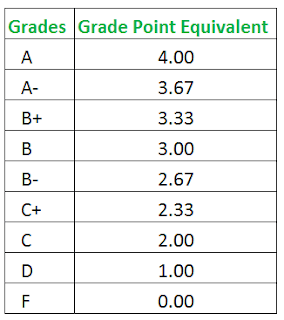# CALCULATE YOUR CGPA MANUALLY BEFORE THE DEPENDABLE AUTO CALCULATOR WILL BE AVAILABLEHow to know your overall status at the end of each semester.
```A - 5 points
B - 4 points
C - 3 points
D - 2 points
E - 1 point

F - 0 point```
*Before we venture into NOUN GPA calculation; Note that:*
70-100= A.
60-69= B.
50-59= C.
45-49= D.
40-44= E.
39 downwards= F.
*Now, let us assume, I did 11 courses and this was how my score went in the 11 courses after calculating scores, with their units in front of them. Let’s assume these were my courses, grades & units;*
1) ECN101 – B. 2unit course
2) TFC116- B. 2unit course
3) ENT121 – C. 3unit course
4) BAT128- C. 2unit course
5) GMB142 – A. 2unit course
6) BRF136- A. 3unit course
7) KFC108- A. 2unit course
8) GEJ158 – D. 3unit course
9) OBJ114 – D. 2unit course
10) IBB131 – E. 2unit course
11) FFK104- F. 2unit course
Now, we have 11 courses, with their grades & units in front of them. Let’s understand the grades;
*A=5, B=4, C=3, D=2, E=1, F=0.*
How to Calculate NOUN GPA
*Now, let’s start with the first course ECN101 for NOUN GPA calculation.*
I scored B in ECN101 above. Since B= 4.
I’ll multiply 4 by the unit of the course, which is a 2unit course.
So; 4 x 2unit= 8. So for ECN101 = 8.
Next is LAW116, I scored B as well. Since it is a 2unit course, I’ll calculate 4 x 2units= 8. So, TFC116 = 8.
Next is ENT121, it was a C. C = 3, which is a 3 unit course. So, calculation will be; 3 x 3units= 9. ENT121= 9.
Next is BAM128, I scored C, which is a 2unit course. The calculation will be; 3 x 2units= 6. BAT128= 6.
Next is BUS142, I scored A. A=5, which is a 2unit course. So; 5 x 2units= 10. GMB142= 10.
Next is BIO136, I scored A, which is a 3unit course. So; 5 x 3units= 15. BRF136= 15.
Next is CHM108, I scored A, which is 2unit course. So: 5 x 2units= 10. KFC108 = 10.
Next is GEO158, which is a 3unit course, I scored D, D = 2. So; 2 x 3units= 6. GEJ158= 6.
Next is CHM114, which is a 2units course, I scored D. So; 2 x 2units= 4. OBJ114= 4.
Next is ECO131, which is a 2units course, I scored E. E = 1. So; 1 x 2units= 2. IBB131= 2.
Next is AGR104, which is a 2unit course, I scored F. F = 0. So; 0 x 2units= 0. FFK104=0.
Now, having done all the 11 courses calculation, we will have to finalized it by adding all the course calculations together. So that will give us;
*8 + 8 + 9 + 6 + 10 + 15
+ 10 + 6 + 4 + 2 + 0 = 78.*
Now, add the total units of all the course together;
*2 + 2 + 3 + 2 + 2 + 3 + 2 + 3 + 2 + 2 + 2 = 25.*
*_So, divide the added course calculation by total units._*
Which is; 78 ÷ 25 = 3.1.
So, there you have it, your NOUN GPA is 3.1. That is 2nd class lower.
*N:B: Results showing incomplete, due to NO EXAM or any other reason will not be calculated - kindly neglect it. All you'll have to do for next semester is register for exam again and rewrite.*
*NOUN GPA Classification:*